## research papersJOURNAL OFSYNCHROTRONRADIATION
ISSN: 1600-5775
Volume 26| Part 1| January 2019| Pages 109-118

## Investigation of glitches' in the energy spectrum induced by single-crystal diamond compound X-ray refractive lenses

aDepartment of Mathematics and Physics, University of Stavanger, 4036 Stavanger, Norway, bEuropean Molecular Biology Laboratory, Hamburg Unit, Building 25a, Notkestrasse 85, Hamburg, Germany, cThe X-ray Optics and Physical Materials Science Laboratory, Immanuel Kant Baltic Federal University, Kaliningrad, Russian Federation, dDepartment of Physics, Norwegian University of Science and Technology, Trondheim, Norway, and eEuropean Synchrotron Radiation Facility (ESRF), BP 220, 38043 Grenoble, France
*Correspondence e-mail: qiuyuan.zhang@uis.no, mpolikarpov@embl-hamburg.de

(Received 29 August 2018; accepted 19 October 2018)

Single-crystal diamond stands out among all the candidate materials that could be exploited to fabricate compound refractive lenses (CRLs) owing to its extremely stable properties. Among all related experimental features, beam divergence, χ-angles relative to the incoming beam in Eulerian geometry and different positions of the X-ray beam relative to the lens geometry may influence the transmission energy spectrum of CRLs. In addition, the orientation of the single-crystal diamond sample may also affect the glitches significantly. To verify these initial assumptions, two experiments, an energy scan and an ω-scan, were set up by employing a polished diamond plate consisting of five biconcave lenses. The results show that beam divergence does not affect the spectrum, nor do χ-angles when ω is set to zero. Nevertheless, different incident positions have an appreciable effect on the transmission spectrum, in particular the strengths' of the glitches. This is attributed to absorption. The ω-scan setup is capable of determining the so-called orientation matrix, which may be used to predict both energy positions' and strengths' of the glitches.

### 1. Introduction

Since synchrotron radiation was observed in 1947, it has been proven very powerful in probing the inner structure of matter. Implementation of synchrotron facilities results in state-of-the-art fundamental and applied research, and synchrotron radiation facilities have sprung up worldwide. The European Synchrotron Radiation Facility (ESRF) and PETRA III are two successful examples of high-class third-generation synchrotron sources. However, scientists are still in pursuit of more brilliant sources, which promotes the construction of the fouth-generation synchrotron facilities, such as the implementation of MAX IV (Austin et al., 2018) and completion of the ESRF upgrade (Chenevier & Joly, 2018).

In pace with the more and more brilliant X-ray sources, advances in the corresponding X-ray optics are also progressing rapidly. Among all the branches in X-ray optics, focusing optics play an important role, because focused X-ray beams have higher intensity gain and are smaller in size, and thus they are preferable for both diffraction and imaging. Several types of X-ray focusing devices have come into operation, such as compound refractive lenses (CRLs). CRLs were demonstrated for the first time by Snigirev et al. (1996) in the form of 31 drilled cylindrical holes in an aluminium block, which led to a revolution in this area and attracted worldwide attention. Due to the X-rays' high frequency, anomalous dispersion (Als-Nielsen & McMorrow, 2011) takes place as more resonances are passed and the refractive index for the X-rays is thus slightly smaller than unity. The index of refraction is written as n =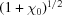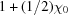=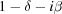, where χ0 is the average electric susceptibility, δ characterizes the refractive decrement and is of the order of 10−6, while β characterizes the absorption coefficient and is of the order of 10−9 far from any absorption edge. This refractive decrement is so small that a stack of concave lenses with radii of 50–500 µm should be used to achieve optimal focusing effect and meter-scale focal length. The described CRL-design makes refractive lenses easy to use, versatile, compact and robust X-ray optical devices which has been proven by the variety of their applications such as microfocusing, coherent microscopy, interferometry and beam conditioning. A diversity of lens types, shapes and forms also led to development of lens-based devices, with the most frequently used one being called a transfocator' (Vaughan et al., 2011; Zozulya et al., 2012) that has an adjustable number of mechanical cartridges with lenses inside.

The choice of lens material becomes a very important issue in light of modern X-ray sources with increased brilliance. In order to reduce the lens contribution to speckles and wavefront distortions, X-ray refractive optics tests new materials as alternatives to polycrystalline beryllium, which is currently the most frequently used for lens manufacturing. For instance, diamond lenses (Snigirev et al., 2002; Terentyev et al., 2015, 2017; Polikarpov et al., 2016a,b) were introduced in both planar and rotational parabolic shapes. They were made of single-crystal diamond, which is considered as an ideal material because it does not cause any undesired diffuse scattering, and at the same time withstands high heat-load induced by synchrotron radiation. Being versatile for a wide range of applications, diamond CRLs, however, have a drawback for spectroscopic experiments: recent published scientific articles have noticed a so-called glitch effect' (Polikarpov et al., 2018) in the energy spectrum. Essentially, glitches appearing in the normalized transmission spectrum represent a drop of intensity and may in general be attributed to many sources, such as multiple diffraction, extinction and Bragg diffraction. Among all these factors, Bragg diffraction usually plays a dominant role, and is the main focus in this paper.

Even though glitches always appear in the transmission spectrum of single-crystal materials, there should be approaches to design a desired experiment in order to minimize glitch influence. To do so, any experimental features that may affect the glitches need to be investigated carefully. Bragg diffraction usually plays a dominant role and it is thus necessary to calculate the so-called orientation matrix'. Via this orientation matrix, one should be able to obtain the crystal orientation with respect to the incident X-ray radiation as it governs the prediction of glitch positions caused by Bragg diffraction. A full understanding of the above issues requires both experimental observations and theoretical modeling of glitch behavior under various conditions. This will be the main focus of this paper. We will present experimental results obtained at the BM31 ESRF beamline, where we investigated the influence of beam size, beam divergence, lens parameters and lens orientation on the glitches induced by the single-crystal diamond CRLs.

### 2. Samples and experimental setups

#### 2.1. Single-crystal diamond refractive lenses

The diamond refractive lenses tested in the present study were described in great detail by Polikarpov et al. (2015). They were fabricated by Nd-YAG laser in the commercially available single-crystal diamond plate supplied by Element Six Ltd (Ascot, UK; product reference No. 145-500-0248). The approximate edge orientation with respect to the beam is 〈100〉 with 3° miscut uncertainty. The diamond plate has two sets of CRLs, i.e. CRL5 and CRL2. Note that the subscript here indicates the number of biconcave parabolic lenses. The experimental results in this paper were obtained by impinging synchrotron radiation on one set of lenses, CRL5 (cf. Fig. 1).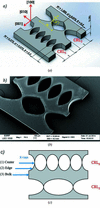Figure 1 (a) Sketch of the diamond lenses, with the red arrows showing the approximate crystallographic directions. W, d and R correspond to the width, web size and radius at the parabola apex of the lens, respectively. All dimensions are in millimetres. (b) Scanning electron micrograph of the diamond plate with refractive lenses. (c) Impingement of the X-rays with the (1) center, (2) edge and (3) bulk material of the diamond lenses (from top to bottom). The notion of bulk material' refers to the part with no profiling between CRL5 and CRL2.

#### 2.2. Experimental setup

This experiment was set up at one of the Swiss–Norwegian Beamlines (SNBL), BM31. This is a bending magnet beamline of the ESRF and it specializes in high-resolution powder diffractometry and EXAFS spectrometry. The beamline is equipped with two monochromators: a Si(111) channel-cut monochromator supplies fast energy scans over a wide range of energies, while a cryogenically cooled Si(111) double-crystal monochromator is normally used for the diffraction measurements. A sample can be mounted on the three-cradle goniometer, with three translational and three rotational axes to determine crystal orientations. Fig. 2(a)shows the experimental hutch of the BM31 station to give the reader an intuitive understanding of the experimental layout, while Fig. 2(b)is a schematic drawing of the three-cradle goniometer. The rotation of χ is realized by using the goniometer with driven motors, and the positive sense of χ-rotation is counterclockwise when viewed from the X-ray synchrotron source with ω = 0°. Following Hamilton's definition (Sands, 1982), the positive sense of ϕ rotation is counterclockwise when viewed from the top of the circle. Likewise, the positive direction of ω rotation is anti-clockwise.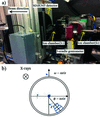Figure 2 (a) Some key instruments of the experiment, e.g. MAR345 detector, two ionization chambers, three-cradle goniometer. (b) Schematic representation of the goniometer axes with available rotations in Eulerian geometry. Arrows corresponds to positive directions of rotation.

The intensity of X-ray radiation can be measured with ionization chambers I0 (upstream of the sample stage) and I1 (downstream of the sample stage). In addition, a MAR345 detector is installed for orientational determination. The MAR detector is an image-plate detector (2D detector) which can be used to visualize and record diffraction patterns from the sample. It features a large active area and high sensitivity, which enables data collection time to be reduced and high-quality diffraction data to be obtained.

Figs. 3(a) and 3(b)show schematically the two experimental setups that we used for the experiment described in the present paper. These two setups combined will help us have a thorough understanding of the glitches in the transmission spectrum.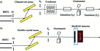Figure 3 Layout of the experiments at BM31, SNBL. The arrows in both figures specify that the X-ray beam propagates from left to right. Panel (a) depicts schematically the energy scan with/without a condenser. Panel (b) is the fixed-wavelength ω-scan setup which is used for orientational measurements. The slit size of S2 is 126 µm × 400 µm (horizontal × vertical).
##### 2.2.1. Energy scan setup

In the energy scan mode [Fig. 3(a)], the goal was to measure the transmission spectrum and investigate how some experimental features, e.g. beam divergence, χ angles in Eulerian geometry and different incident positions of the X-rays on diamond CRLs, may influence the appearances of the glitches at some specific energies. Therefore, the experimental setup employed the channel-cut monochromator to perform fast scanning continuously in the user-defined energy interval. The slit S2 was used to modify the beam cross-section. To measure the intensity of X-ray radiation in a transmission geometry, we used two gas-filled ionization chambers, I0 and I1, that were mounted upstream and downstream of the sample holder, respectively. The diamond sample was installed on the three-cradle goniometer which was aligned so that the beam hit the center of the sample.

Although the third-generation synchrotron source has very high spatial coherence (Snigirev et al., 1995), Cloetens et al. (1997) pointed out that it still could be viewed as a very small spherical source with certain divergence due to the very long source-to-sample distance (∼50 m). It is thus expected that the beam divergence may influence the transmission spectrum. To verify this, the condenser consisting of five beryllium lenses was placed upstream of the I0 chamber to change the beam divergence. These beryllium lenses are for practical purposes identical, and each of them has a radius of curvature of 50.0 µm at the apex and center thickness of 29.7 µm. The focal length of this condenser is 1.5 m at E = 10 keV and 6.5 m at E = 20 keV, generating a divergence of 170 µrad and 40 µrad, respectively.

##### 2.2.2. ω-scan setup

The ω-scan setup [Fig. 3(b)] was intended for calculation of the sample orientation from the recorded diffracted patterns. Unlike the energy scan setup, we used the double-crystal monochromator to keep the wavelength of X-ray radiation fixed. We also did not use the condenser and ionization chambers, so, after passing the collimation slit (S2), X-rays arrived at the three-circle goniometer with a sample mounted so that the X-ray beam hit its center in Eulerian geometry. Further downstream, the MAR345 detector was used to record diffraction patterns during the scan of the sample through the ω-axis.

Before the ω-scan of the diamond lens, the setup was calibrated by employing a Standard Reference Material (SRM), LaB6 660c. This is vital because we could deduce some key instrumental parameters, such as sample-to-detector distance, wavelength of the radiation and center positions of the incoming beam, precisely.

During the ω-scan of the diamond lens, the angular range was limited from −49° to +20° to guarantee that the goniometer did not collide with other inherent beamline equipment. It should be borne in mind that each frame in the dataset records all occurrences of diffracted intensities in an angular interval of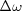= 1°, i.e. an integration frame. Each frame took approximately 10 min, including ∼5 min recording and ∼5 min readout time by laser. In combination with the instrumental parameters from the calibration process, the collected images from the MAR detector would help determine the orientation matrix (also known as UB matrix'). This will be discussed in depth in the following sections.

#### 2.3. Data processing

Special care should be taken in order to identify whether the glitches are from the monochromator or the diamond lenses. In other words, the glitches from the monochromator may accumulate and are seen by both chambers. Therefore, an appropriate data processing method was chosen to cancel out the monochromator's glitches. The concrete steps are summarized as follows: first, we divided I1 by I0 (I1/I0) as a function of the incident energy and masked all the glitches in this data column. Then, low-order polynomial (1 or 2, maximum 3) fitting through the remaining I1/I0 line was applied. The purpose of this fit was to mark the 100% reference line and the dips of the glitches were then given as a percentage with respect to the 100% baseline.

The method discussed above is to extract the baseline and then the dip of intensity is obtained with regard to this. The spectra of I0 and I1/I0 shown in Appendix Amanifest that only diamond-induced glitches remain after processing with this method. By importing a raw dataset into the software Origin (OriginLab, Northampton, MA, USA), we could plot the transmitted intensity as a function of the X-ray incident energy.

### 3. Implementation of the orientation matrix (UB matrix)

The purpose of the ω-scan setup is to predict the positions' and strengths' of the glitches and then subsequently avoid them at some specific energies. This would not be possible without knowing the orientation matrix (hereafter called the UB matrix' for simplicity). Therefore, one needs to calculate the UB matrix which establishes the relations between the crystallographic orientation of the sample relative to the incoming beam.

The UB matrix was first proposed by Busing & Levy (1967) to cater for the increasing demands from three- and four-circle X-ray or neutron diffractometer user groups. As has been discussed in their work, the UB matrix is, in practice, a product of two matrices and can be computed by multiplying the orthogonal rotation matrix U with the orthonormalization matrix B. The procedure on how to calculate the UB matrix has been given in Appendix B.

Once the UB matrix is evaluated according to a certain procedure, a new quantity, g, is introduced to characterize the orientation of [−1 0 0] in the laboratory system quantitatively: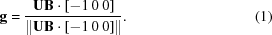It is worth noting that the prerequisite that the direction of the incoming beam is in perfect alignment with g should be satisfied.

By applying the conversion between the energy (in keV) and the wavelength (in Å), the X-ray energy leading to Bragg diffraction is then given by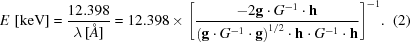In this equation, h = [hkl] represents the coordinate matrix and G−1 is the reciprocal metric tensor. By skipping extinction reflections and negative energy solutions based on selection rules for diamond, we could predict the energy positions where glitches may occur and their corresponding kinematical structure factors within the pre-set energy range.

With the availability of relevant information from the ω-scan, we are able to calculate the UB matrix. Given this UB matrix, the real orientation of the diamond lenses with respect to the X-ray radiation can be readily obtained and then used as an input parameter to predict the glitch positions and corresponding kinematical' strengths. This may help us have a better understanding of the transmission spectrum and avoid the glitches at some energies.

### 4. Results and discussion

To start with, we decided to study the influence of the divergence on the glitches. The overall energy scan range was limited to 10–20 keV with a selected interval of 1 eV. In order to increase the signal-to-noise ratio, the energy scans were repeated three times from 16 keV to 18 keV for various experimental conditions. These curves were then averaged and are shown in Figs. 5(a) and 5(b). Note that all the figures below have the same scaling.

By comparing Figs. 4(a) and Fig. 4(b), it appears that the two spectra are in agreement on the presented scale in the two different situations: without and with a condenser. Beam divergence, at least, does not play an important role in the energy spectrum, but whether it can be evaluated as a minor factor still remains to be verified. In order to assess this, the direct overlay of the four strongest glitches on an even finer scale is shown in Fig. 5.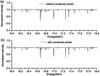Figure 4 Plots of intensity versus energy: (a) the X-ray impinges on the center of CRL5 without any condenser; (b) the X-ray impinges on the center of CRL5 with a condenser placed upstream of the sample stage.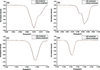Figure 5 The four graphs show direct overlays of the four strongest glitches on a finer scale.

As expected, the four graphs in Fig. 5show that the glitches become slightly wider as the beam divergence is increased. However, the influence of beam divergence is very minor and could be associated with focusing ability provided by the condenser.

The next step was to evaluate how different χ angles may influence the glitches in the transmission spectrum. To do so, we moved the χ-cradle to 15° and 30° counterclockwise relative to the zero position while keeping the other two cradles fixed at the zero position. All three glitch patterns in Fig. 6are quite similar except for some minor differences in both glitch positions and strengths. In this particular case these minor effects are most likely due to minor deviations from the ideal alignment. During the whole rotation process, small misalignments may be further expanded because they may generate corresponding components in other cradles and make glitch patterns with different χ-angles differ slightly. Polarization issues may also influence the glitch strengths, but, for the accessible χ-angles interval, such effects are small. To conclude, the influence on the glitch pattern positions owing to rotation around the χ shaft is only considered negligible if the sample is perfectly or quasi-perfectly aligned.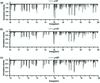Figure 6 Transmission spectra of different χ angles relative to the incoming beam when two other cradles are at zero positions. From top to bottom the transmission spectra are for χ = 0°, χ = 15° and χ = 30°.

Another possible experimental feature is different scanning positions. The incident X-ray beam was confined to interact with different parts of the lenses and their corresponding spectra are plotted in Fig. 7. It can be seen that all the glitches appear at the same energy positions but differ significantly in strengths: an X-ray traversing the center of the lenses has the smallest dips of glitches while that passing through the bulk part of the material has the largest drops in intensity. The glitches show strong dependence on the thickness of the sample, which is due to the absorption process. In other words, different incident positions can influence the glitch patterns significantly, especially the dips of the glitches.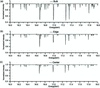Figure 7 Transmission spectra by confining the X-rays to interact with the (a) bulk, (b) edge and (c) center of CRL5.

The goniostat permits rotations around certain shafts in Eulerian geometry and collects a sequence of adjacent non-overlapping rotation images from the diamond sample at a fixed X-ray wavelength. Only a few reflections are, however, accessible for the given setup because 2θmax here is approximately 30°.

The actual orientation of [−100] with respect to the laboratory system is calculated to be 3.2° from equation (12). An example of the theoretical predictions with the corresponding experimental results is visualized in Fig. 8. It should be noted that the heights for the two predicted sets represent strengths' of the glitches, which are estimated by introducing the squared kinematical structure factors of the actual reflection appearing.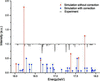Figure 8 Simulated figures with and without the orientational correction, together with experimental results, are plot in the same figure for comparison. The black curve is the normalized intensity retrieved from experimental data (X-ray radiation interacts with the center part of CRL5 without a condenser), while the red and blue vertical lines represent the squared kinematical structure factors (scaled) without and with orientational correction, respectively.

From Fig. 8we notice that only six theoretical diffraction events occur within the energy interval in question for a situation without orientational correction (red lines). It is thus advantageous to manufacture the lenses with a preferred alignment; however, the degree of miscut' will influence the results depending on the possibilities for additional alignment-mechanisms for individual lenses, or lens-stacks. In comparison, many more reflections (35 glitches in total) are brought in by introducing the orientational correction, more in line with the observation (35 glitches) from the real experiment. This means the theoretical prediction without correction does not match with the experimental data at all and should be considered invalid. However, the theoretical glitches' with correction (blue lines) are the same in number as those appearing in the experiment. Nevertheless, we note that there are still some discrepancies between the experimental and theoretical glitch positions. This may be attributed to the uncertainty associated with the actual UB matrix. For instance, in the ω-scan, each image collected by the detector records all occurrences of diffracted intensities within a 1° angular range, which means that the ω-angle where Bragg reflections occur is hampered with an event, and thus introduces an uncertainty in calculating the UB matrix. A more accurate Bragg angle could for instance be obtained by replacing the MAR detector with a point detector in the 1° angular range, which would lead to an even better prediction of the glitch positions. In spite of this, it is still necessary to introduce the orientational correction because this may help predict the glitches much more accurately and avoid them efficiently. To summarize, the result shows significant sensitivity of predicted positions to the sample orientation, and the prediction with orientational correction shows more profound agreement with the experiment compared with that without applying any correction.

### 5. Conclusion

In the present paper we showed how various experimental conditions may affect the positions and strengths of glitches. We found that the spatial position of the incident X-ray beam (relative to the lens aperture) has the strongest effect on the strength of glitches. This is mainly because of the absorption process, practically meaning that glitches are becoming more pronounced with the increase of the thickness that X-rays pass through. Therefore, one needs to consider minimizing the longitudinal thickness of the diamond lenses under experimental conditions where the presence of glitches is critical. This could be achieved by using diamond lenses with larger parabola radii. Another possible solution would be to use only the central part of the lens aperture where it has the minimal thickness of the material.

The results also show that beam divergence has very limited influence on the transmission spectra. This practically means that diamond lenses can be installed in different optical schemes of glitch-sensitive applications while dealing with various geometries and distances at the modern synchrotron beamlines.

Another important result presented is a theoretical model which has been proven consistent with the experimental results. We believe this model to be useful as it allows one to predict glitch positions and strengths if the crystallographic orientation relative to the X-ray beam is known to an adequate precision. Therefore, by implementing our calculations for the accurately positioned single-crystal lens with a known orientation, we are able to select specific energy intervals where the glitch effect has the minimal impact. This will make it possible to deliver a more stable and uniform incoming beam in spectroscopy-like experiments with diamond lenses. Additionally, our model is not restricted to single-crystal diamond CRLs only, but can also be directly applied to other transmission diamond devices, e.g. time-of-flight neutron diffraction employing diamond anvil cells (Guthrie et al., 2017).

To conclude, the experimental and theoretical results presented above are complementary for refractive lenses made of different single-crystal materials (for example, silicon), leading to better understanding of their performance at modern synchrotron sources.

### Transmission spectra of I0 and I1/I0

Fig. 9aims to demonstrate that glitches from the monochromator have been canceled out successfully. In order to achieve a better resolution, a small energy range between 19 keV and 20 keV is chosen. The transmission spectrum plot in red symbolizes the normalized intensity recorded by the I0 chamber, while the black one is from I1/I0. By comparing these two patterns, we can see that the small red glitches caused by the silicon monochromator do not appear in the black transmission spectrum. In conclusion, the glitches in the transmission spectrum after processing are purely induced by the diamond samples and our processing method is thus considered valid.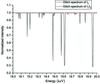Figure 9 Transmission spectra retrieved from I0 and I1/I0. The red curve records the glitches induced by the silicon monochromator, namely I0, while the black curve shows the glitches after data processing (I1/I0).

### Procedures for calculating the UB matrix

As has been stated above, the UB matrix is composed of the orthogonal rotation matrix U and the orthonormalization matrix B. The following paragraphs will provide the procedure in greater detail.

The B matrix is an upper triangle matrix which contains information about the unit-cell parameters. Here we will exclusively use the parameters of the reciprocal lattice rather than the direct lattice since Bragg diffraction is a main cause of the glitches. In the systematic scanning process, the most convenient way of constructing the orthonormal coordinate system is to let its x-axis coincide with the reciprocal unit cell vector a*. The y-axis is then defined so that it lies in the a*b* plane and is perpendicular to the x-axis at the same time. The z-axis is chosen to complete a right-handed Cartesian system, which means it is perpendicular to the a*b* plane. Essentially, the B matrix specifies the components of the unit cell of the reciprocal lattice in the Cartesian laboratory coordinate system and has been introduced by Giacovazzo et al. (2002),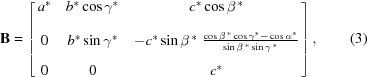a*, b*, c* are the conventional reciprocal lattice parameters and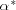,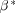,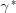are the included angles betweenand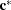,and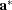,and, respectively. Diamond has a cubic lattice structure in direct space, so it is deduced to possess a cubic lattice in reciprocal space based on the definition of reciprocity. Therefore, in the case of diamond, the B matrix can be reduced to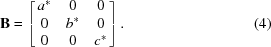The purpose of the B matrix is to transform from the reciprocal lattice vector, denoted as h, to the Cartesian coordinate system with respect to the crystal and could be described by the following formula: hc = Bh, where hc is the scattering vector in the crystal Cartesian system and represents a vector normal to a family of crystal lattice planes h. In order to find the Bragg reflections induced by these planes, another rotation matrix, denoted as the U matrix, is also needed.

The U matrix is an orthogonal rotation matrix (UT = U−1) which transforms from the orthonormal coordinate system attached to the crystal to another Cartesian coordinate attached to the laboratory system.

In essence, the UB matrix acts on a reciprocal lattice vector h and brings into coincidence with the scattering vector,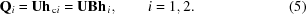In this equation, the subscript i = 1, 2 is used to distinguish two types of Bragg reflections.

In order to further show the implications of the UB matrix, the Ewald construction is particularly useful. The wavevector of the incident X-ray radiation k0 is parallel to the x-axis and the outgoing wavevector is parallel to kf; the scattering vector Qi is defined as Qi = kfk0, shown in Fig. 10. An arbitrary reciprocal lattice node is expressed as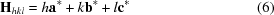where h, k, l are Miller indices. The Bragg condition is satisfied when the scattering vector is equal to the reciprocal lattice vector, i.e. Hhkl = Qi. For the convenience of further calculation, we will define an alignment vector, which is denoted as g0 and is expressed as g0 = −k0 = [−100] in the ideal case. However, the actual incoming beam k′ may slightly deviate from the ideal unit vector of the incoming beam g0 under real experimental configuration, as is illustrated in Fig. 10.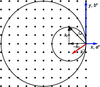Figure 10 Illustration of the Ewald sphere with radius 1/λ for the energy scan, where λ is the wavelength of the X-ray radiation. According to the relation between the energy E and the wavelength, E = hν = hc/λ, the radius of the Ewald sphere is proportional to the energy. Therefore, the internal circle corresponds to the minimum energy and the outer circle corresponds to the maximum energy. Here, −k0 characterizes the direction of ideal alignment while k′ (the red arrow) is the real alignment and deviates slightly from the ideal case. The deviation in this figure is exaggerated.

Before performing further calculations, let us recall the Bragg equation,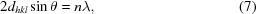where n is an integer (not to be confused with the index of refraction), dhkl is the lattice spacing and is equal to dhkl = a/(h2+k2+l 2)1/2 for a cubic lattice, and θ is the angle between the incident beam and the family of lattice planes with Miller indices h,k,l. Diamond is in the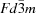space group and possesses a face-centered-cubic Bravais lattice. The allowed reflections should thus satisfy the condition that all Miller indices are odd, or even with h + k + l = 4m (m is an integer). Otherwise, they are so-called forbidden reflections due to extinction. This rule is also very useful in predicting both the energy positions and strength of the glitches.

In this case, two types of reflections are found and they should come from the lattice planes with the two lowest 2θ-angles, namely {111} and {220}. However, this should be verified by comparing the theoretical and actual 2θ-angles. The theoretical 2θ-angles are completely determined from the Bragg equation, recast in the form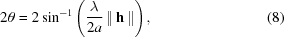where ||h|| is the norm (or positive length) of h and the other symbols have their usual meanings.

In crystallography and materials science, the term `metric' (Prince, 1982) is used to indicate that the metric tensor pertains to the measurement properties of the space. As mentioned before, the sample-to-detector distance and the pixel size of the MAR detector are already known, and thus we could define the measurement metric, which is denoted as M. Following on from that, the metric B is then obtained by performing Cholesky decomposition on the metric M. Then we are able to construct the unit vector along the incoming beam s0 and diffracted beam directions sh(x, y) as follows,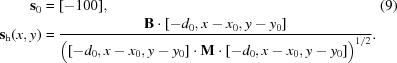In this equation, d0 is the sample-to-detector distance, and x0 and y0 are pixel positions of the direct beam (beam center) on the detector screen. By substituting all the instrumental parameters into equation (7), the actual 2θ-angles can be computed and the types of reflections in principle can be determined.

Another implication of s0 and sh(x, y) is to determine the coordinates of the reciprocal lattice nodes in the Cartesian coordinate system with respect to the crystal. In addition, ω-angles associated with frames which have sharp reflection points should be recorded to compute the U matrix. An ω-rotation matrix R is also needed,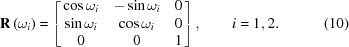Then the coordinates of the reciprocal lattice nodes are expressed as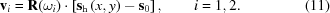In general, two approaches are employed to obtain the UB matrix: (i) using two independent reflections with known lattice parameters; and (ii) using three accessible independent reflections with unknown unit-cell parameters. An important application of approach (ii) is to deduce the lattice parameters of the material from the metric tensor. In equation (9), sh(x, y) stands for the pixel positions of the appearing reflections and is extracted by inspecting all the frames using the software CrysAlis (Oxford Diffraction Ltd, Abingdon, UK) together with their corresponding ω values. The combined use of these parameters will provide an estimation of the UB matrix for this sample, using the procedure outlined in Section 3.

In our case, method (i) is adopted because the lattice parameters for diamond are already known. Here we briefly review the calculations. Ideally, equation (3)should be satisfied. However, due to uncertainties in the angular measurement and lattice parameters, right-handed orthogonal unit vectors, tc1, tc2, tc3, in the Cartesian system with respect to the crystal are defined such that tc1 is parallel to hc1; tc2 lies in the plane of hc1 and hc2, and can be seen as the normalized cross product of hc1 and hc2; tc3 is perpendicular to the planes of tc1tc2. Another triple tφ1, tφ2 and tφ3 based on observations are defined in the same way as tc1, tc2, tc3 simply by replacing hi with vi (i = 1, 2). The orthogonal rotation matrix U is defined such that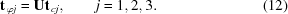Since tcj are orthogonal, the U matrix is deduced to be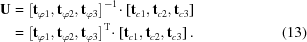Multiplying the U matrix by the B matrix gives the UB matrix.

### Acknowledgements

We gratefully thank PhD candidate Stian Ramsnes from University of Stavanger, Norway, for his kind help in implementing Mathematica 11.3 to predict the glitches with a given UB matrix.

### Funding information

The following funding is acknowledged: Ministry of Education and Science of the Russian Federation (contract No. 14.Y26.31.0002).

### ReferencesJOURNAL OFSYNCHROTRONRADIATION